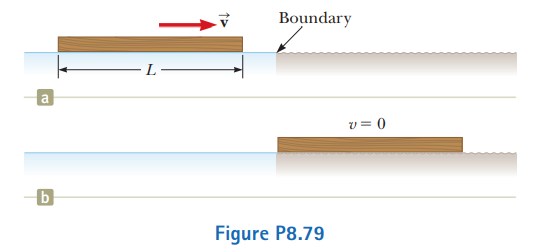# Problem: A uniform board of length L is sliding along a smooth, frictionless, horizontal plane as shown in Figure P8.79a. The board then slides across the boundary with a rough horizontal surface. The coefficient of kinetic friction between the board and the second surface is µk. (a) Find the acceleration of the board at the moment its front end has traveled a distance x beyond the boundary. (b) The board stops at the moment its back end reaches the boundary as shown in Figure P8.79b. Find the initial speed v of the board.

###### FREE Expert Solution

This problem involves kinetic friction force.

Kinetic friction force:

82% (69 ratings)###### Problem Details

A uniform board of length L is sliding along a smooth, frictionless, horizontal plane as shown in Figure P8.79a. The board then slides across the boundary with a rough horizontal surface. The coefficient of kinetic friction between the board and the second surface is µk. (a) Find the acceleration of the board at the moment its front end has traveled a distance x beyond the boundary. (b) The board stops at the moment its back end reaches the boundary as shown in Figure P8.79b. Find the initial speed v of the board.Frequently Asked Questions

What scientific concept do you need to know in order to solve this problem?

Our tutors have indicated that to solve this problem you will need to apply the Energy with Non-Conservative Forces concept. You can view video lessons to learn Energy with Non-Conservative Forces. Or if you need more Energy with Non-Conservative Forces practice, you can also practice Energy with Non-Conservative Forces practice problems.

What professor is this problem relevant for?

Based on our data, we think this problem is relevant for Professor Bentley's class at Adelphi University.

What textbook is this problem found in?

Our data indicates that this problem or a close variation was asked in Physics for Scientists and Engineers - Serway Calc 9th Edition. You can also practice Physics for Scientists and Engineers - Serway Calc 9th Edition practice problems.